# April 29 2009 What do you know about

• Slides: 24
Download presentation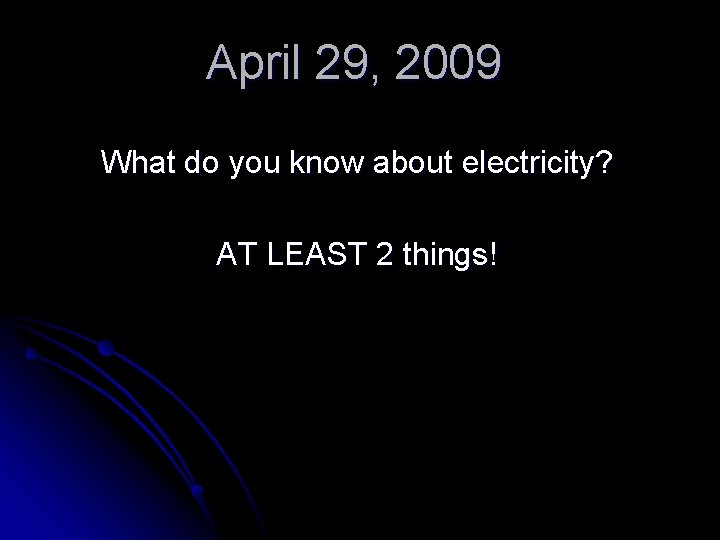April 29, 2009 What do you know about electricity? AT LEAST 2 things!Goal To create working circuits l To define electricity and circuit lQ: What is electricity? l Electricity is the movement of electrons through something (“medium”) l Electrons move for 2 reasons l They are attracted to… PROTONS! (Thanks Paula Abdul!) main reason for electricity! l They are “hit” by another electron and bounce out of the way.Q: What is electricity? Electricity is similar to water moving in a hose.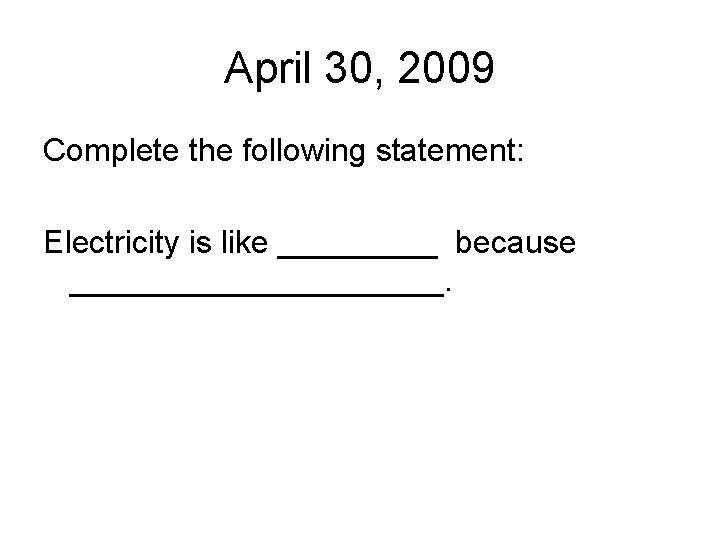April 30, 2009 Complete the following statement: Electricity is like _____ because ___________.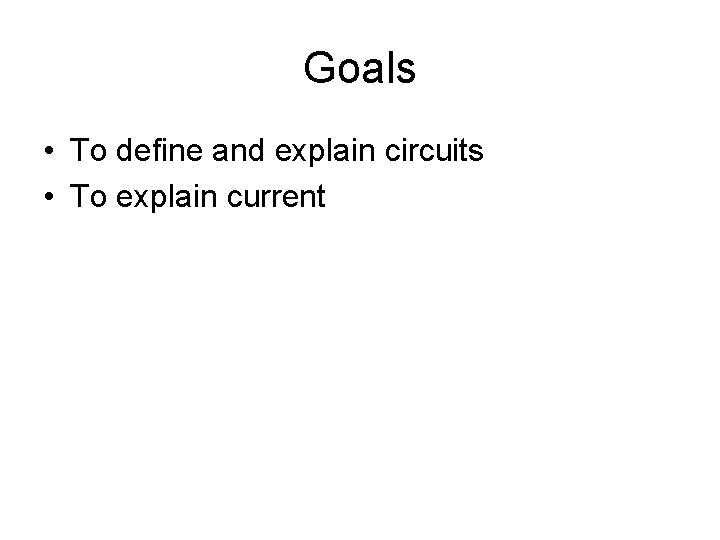Goals • To define and explain circuits • To explain current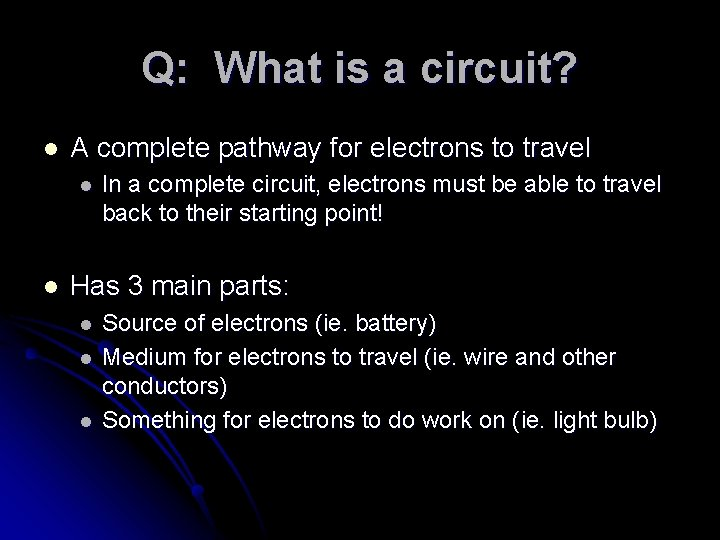Q: What is a circuit? l A complete pathway for electrons to travel l l In a complete circuit, electrons must be able to travel back to their starting point! Has 3 main parts: l l l Source of electrons (ie. battery) Medium for electrons to travel (ie. wire and other conductors) Something for electrons to do work on (ie. light bulb)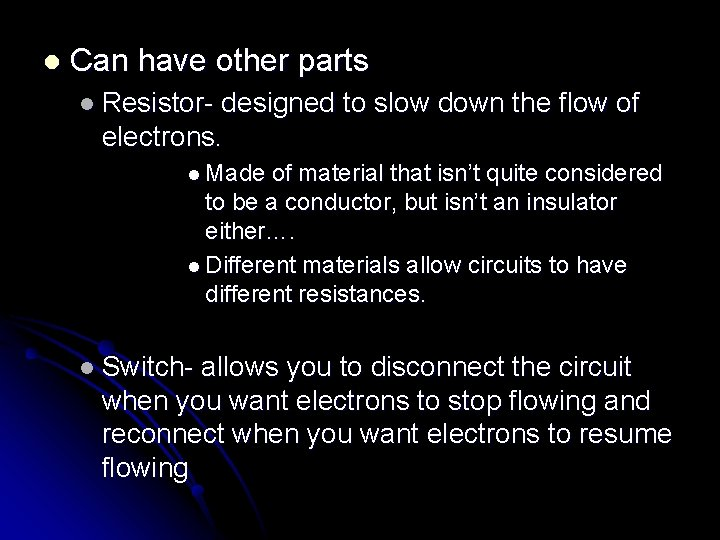l Can have other parts l Resistor- designed to slow down the flow of electrons. l Made of material that isn’t quite considered to be a conductor, but isn’t an insulator either…. l Different materials allow circuits to have different resistances. l Switch- allows you to disconnect the circuit when you want electrons to stop flowing and reconnect when you want electrons to resume flowingQ: What is a circuit? Circuits are often drawn as a diagram using the symbols given below: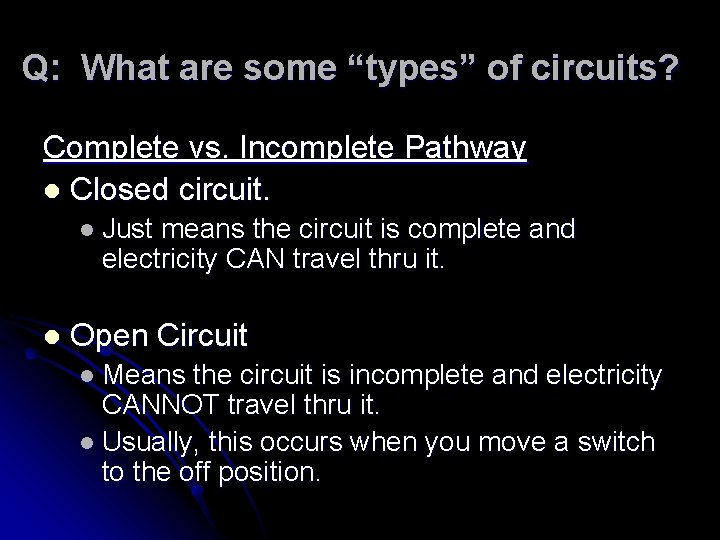Q: What are some “types” of circuits? Complete vs. Incomplete Pathway l Closed circuit. l Just means the circuit is complete and electricity CAN travel thru it. l Open Circuit l Means the circuit is incomplete and electricity CANNOT travel thru it. l Usually, this occurs when you move a switch to the off position.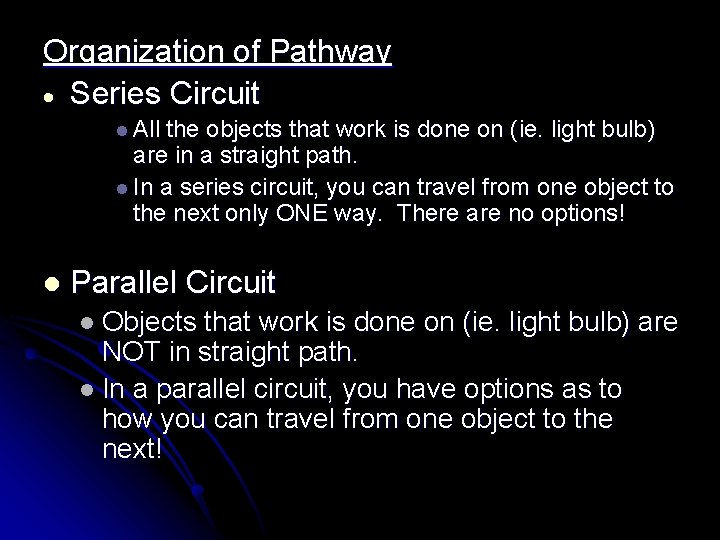Organization of Pathway Series Circuit l All the objects that work is done on (ie. light bulb) are in a straight path. l In a series circuit, you can travel from one object to the next only ONE way. There are no options! l Parallel Circuit l Objects that work is done on (ie. light bulb) are NOT in straight path. l In a parallel circuit, you have options as to how you can travel from one object to the next!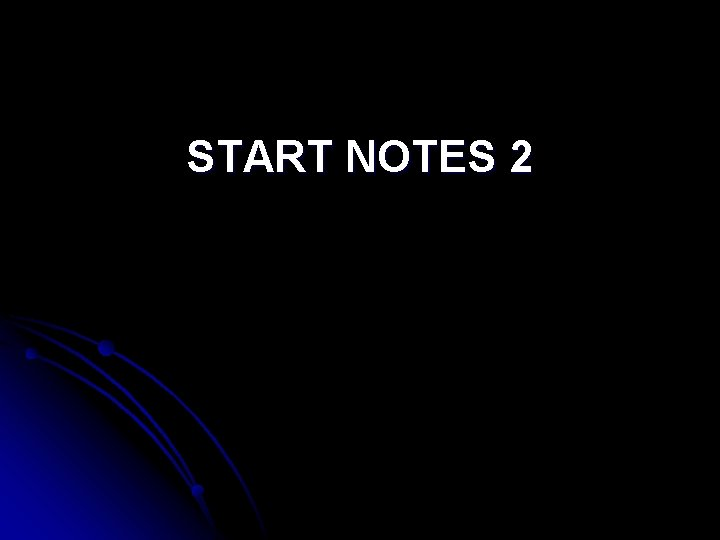START NOTES 2Q: What is an electrical current? Electrons (charges) in motion l Comparison to water hose: This is the moving water l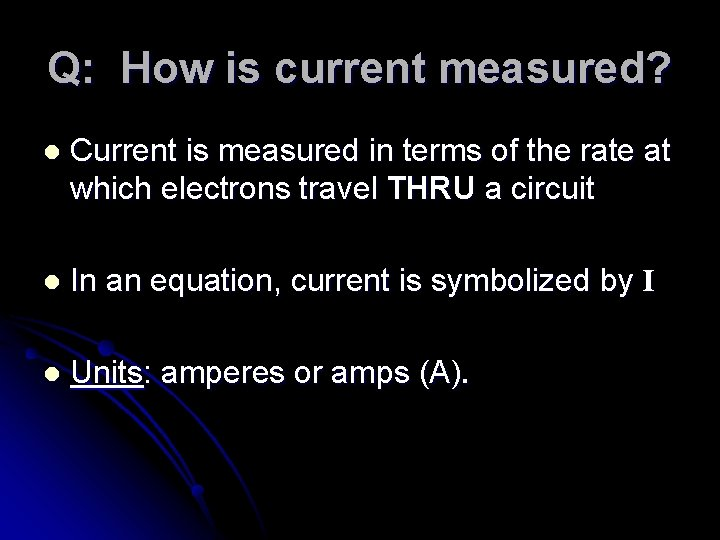Q: How is current measured? l Current is measured in terms of the rate at which electrons travel THRU a circuit l In an equation, current is symbolized by I l Units: amperes or amps (A).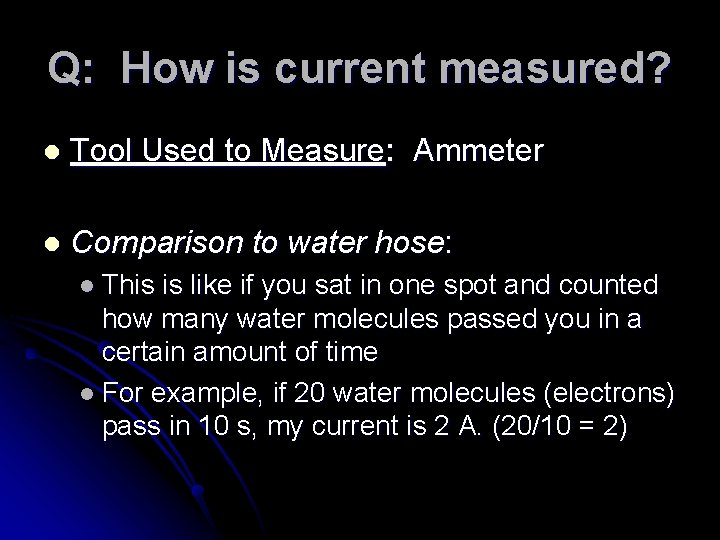Q: How is current measured? l Tool Used to Measure: Ammeter l Comparison to water hose: l This is like if you sat in one spot and counted how many water molecules passed you in a certain amount of time l For example, if 20 water molecules (electrons) pass in 10 s, my current is 2 A. (20/10 = 2)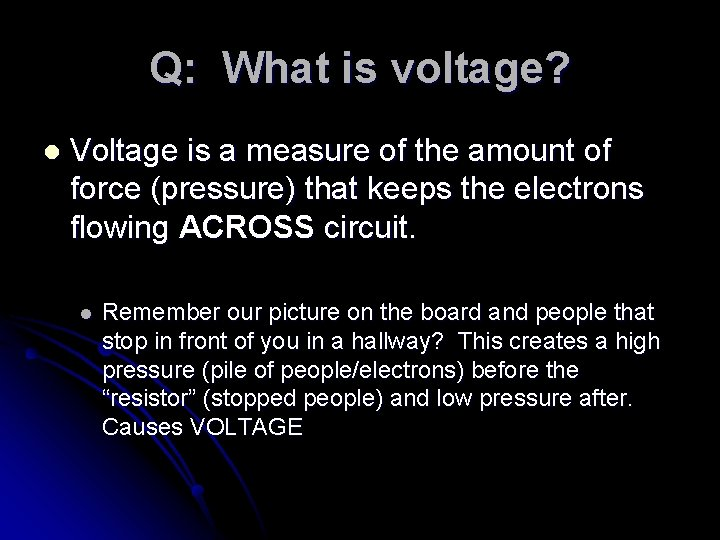Q: What is voltage? l Voltage is a measure of the amount of force (pressure) that keeps the electrons flowing ACROSS circuit. l Remember our picture on the board and people that stop in front of you in a hallway? This creates a high pressure (pile of people/electrons) before the “resistor” (stopped people) and low pressure after. Causes VOLTAGE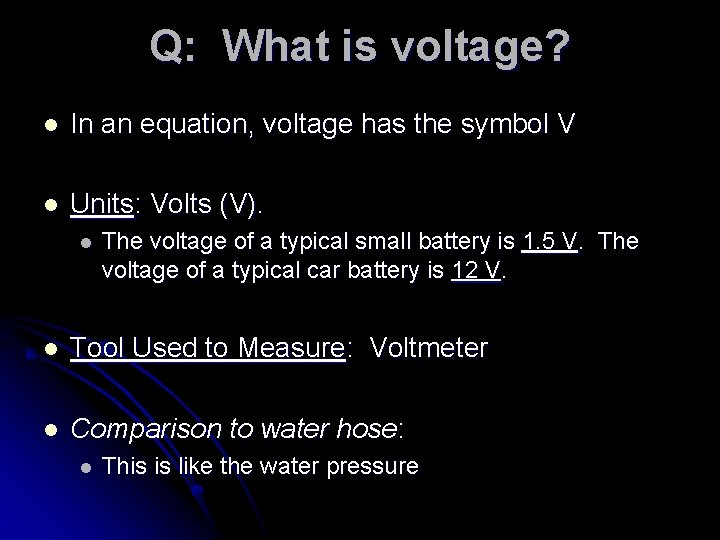Q: What is voltage? l In an equation, voltage has the symbol V l Units: Volts (V). l The voltage of a typical small battery is 1. 5 V. The voltage of a typical car battery is 12 V. l Tool Used to Measure: Voltmeter l Comparison to water hose: l This is like the water pressure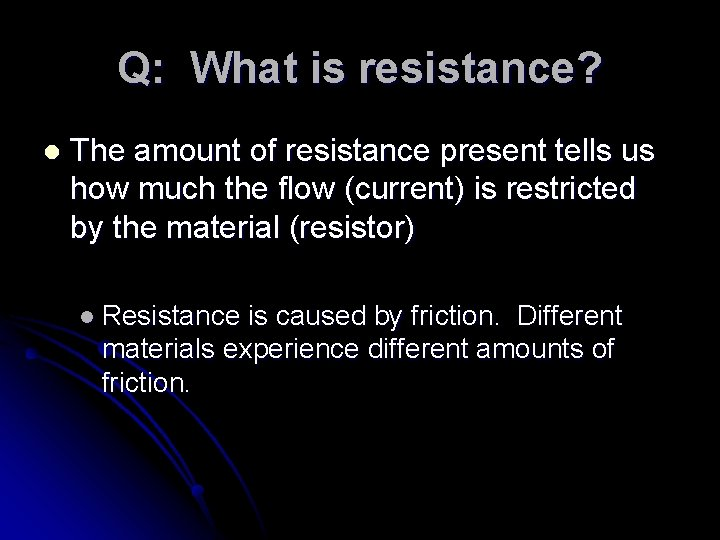Q: What is resistance? l The amount of resistance present tells us how much the flow (current) is restricted by the material (resistor) l Resistance is caused by friction. Different materials experience different amounts of friction.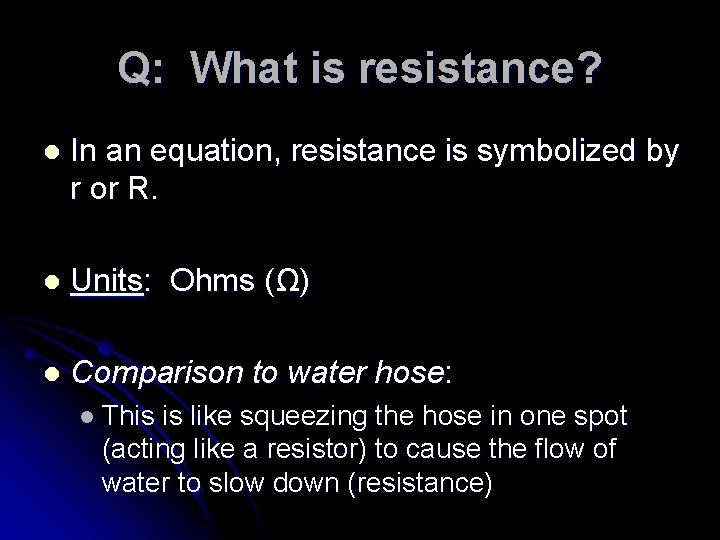Q: What is resistance? l In an equation, resistance is symbolized by r or R. l Units: Ohms (Ω) l Comparison to water hose: l This is like squeezing the hose in one spot (acting like a resistor) to cause the flow of water to slow down (resistance)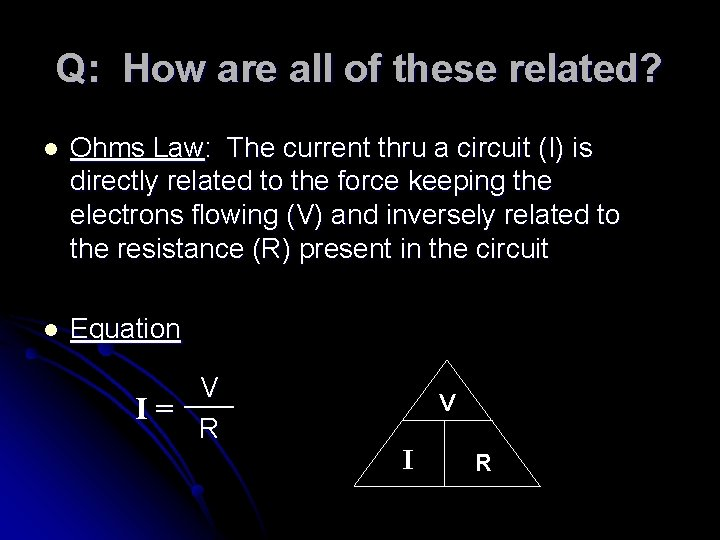Q: How are all of these related? l Ohms Law: The current thru a circuit (I) is directly related to the force keeping the electrons flowing (V) and inversely related to the resistance (R) present in the circuit l Equation V I= R V I R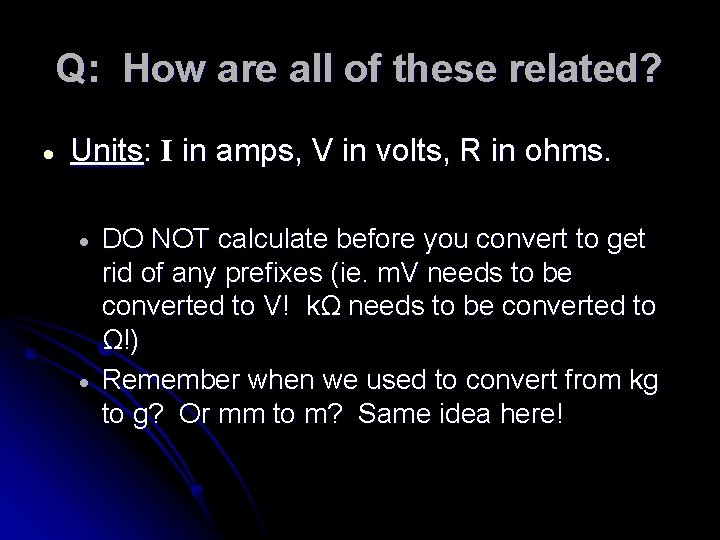Q: How are all of these related? Units: I in amps, V in volts, R in ohms. DO NOT calculate before you convert to get rid of any prefixes (ie. m. V needs to be converted to V! kΩ needs to be converted to Ω!) Remember when we used to convert from kg to g? Or mm to m? Same idea here!1)The headlights of a typical car are powered by a 12 V battery. What is the resistance of the headlights if they draw 3. 0 A of current when they are turned on? R = V/ I = 12 V/3 A R=4Ω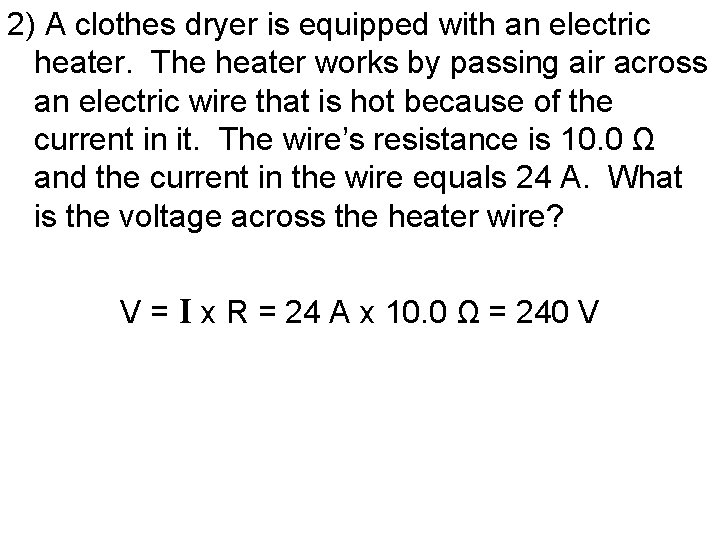2) A clothes dryer is equipped with an electric heater. The heater works by passing air across an electric wire that is hot because of the current in it. The wire’s resistance is 10. 0 Ω and the current in the wire equals 24 A. What is the voltage across the heater wire? V = I x R = 24 A x 10. 0 Ω = 240 V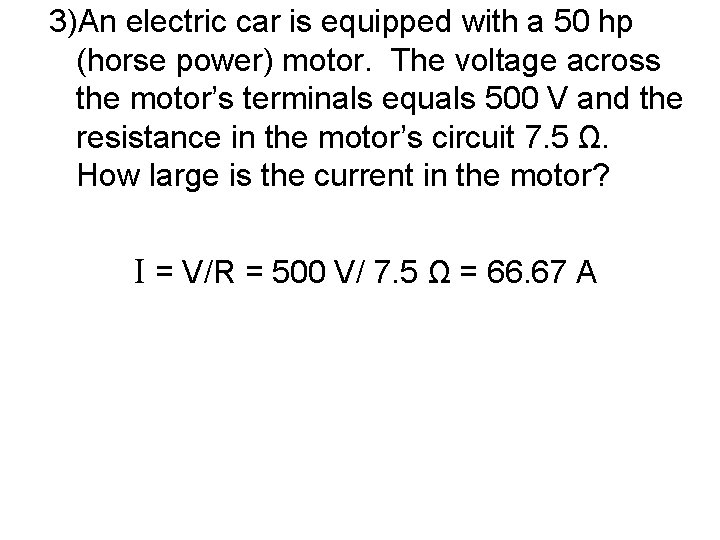3)An electric car is equipped with a 50 hp (horse power) motor. The voltage across the motor’s terminals equals 500 V and the resistance in the motor’s circuit 7. 5 Ω. How large is the current in the motor? I = V/R = 500 V/ 7. 5 Ω = 66. 67 A